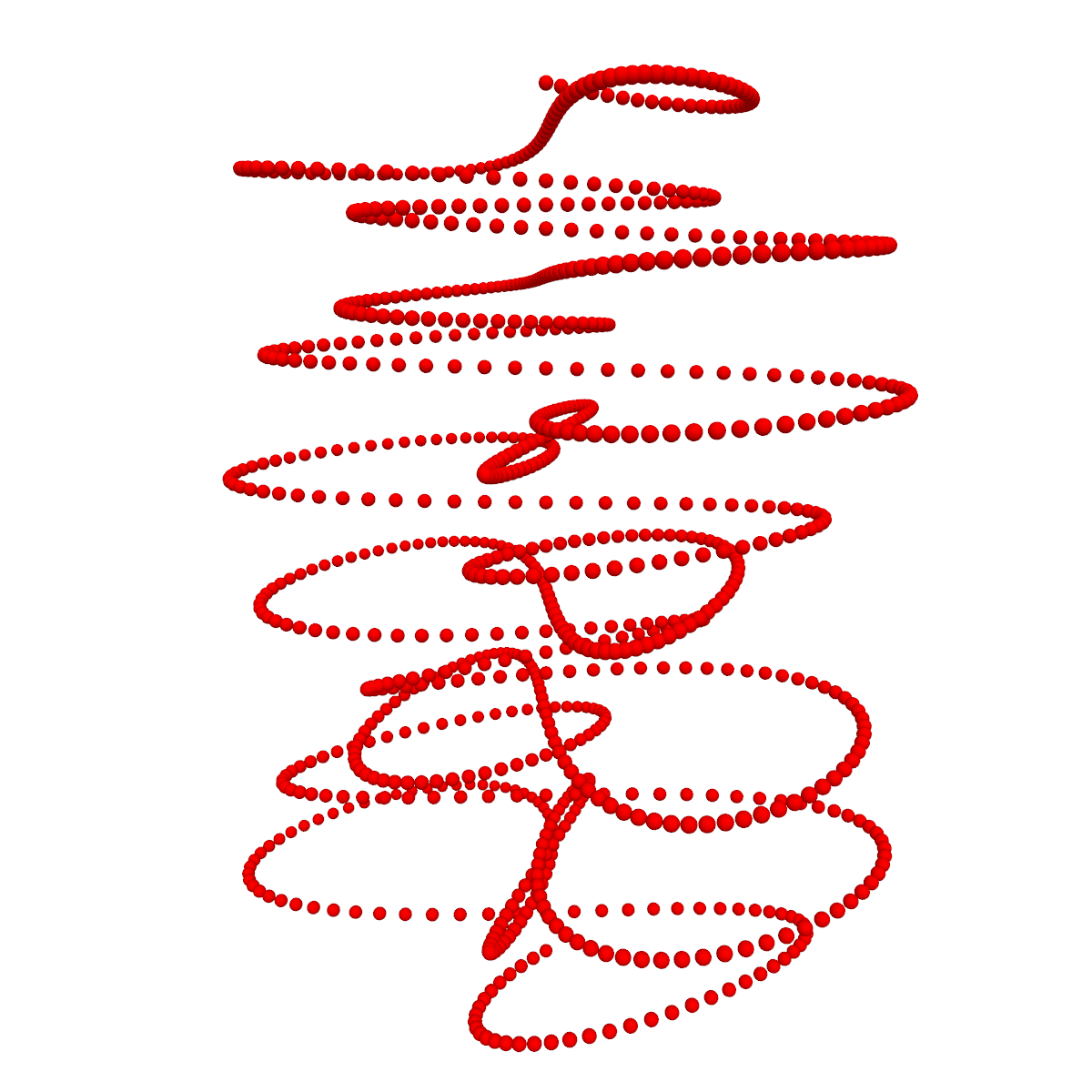﻿ plot – Algosim documentation
Algosim documentation: plot

# plot

The generalised visualisation function.

## Syntax

• `plot(equation[, a, b[, δ]])`

• `equation` is an equation

• `plot(inequality[, a, b[, δ]])`

• `inequality` is an inequality

• `plot(inequalities[, a, b[, δ]])`

• `inequalities` is a chain of two inequalities

• `plot(data)`

• `data` is a sequence of (X, Y) points

• `plot(data)`

• `data` is a sequence of (X, Y, Z) points

• `plot(data)`

• `data` is a sequence of (X, Y, Z, colour, radius) points

## Description

If `equation` is an equation in the Cartesian coordinates `x` and `y` with either the LHS or the RHS equal to one of these variables and the other side not containing this variable, then `plot(equation)` plots this function curve.

Examples:

```y = sin(x)
x = √y + 1
ln(x) + 4 = y```

If `inequality` is an inequality in the Cartesian coordinates satisfying the same restrictions, then the implied planar region is plotted.

Examples:

```y < sin(x)
x > √y + 1
ln(x) + 4 < y```

If `inequalities` is a chain of two inequalities in the Cartesian coordinates `x` and `y` and at least one side is such a variable isolated and the remaining sides functions of this variable, then the implied region (the region between two function curves) is plotted.

Examples:

```sin(x) < y < 2⋅sin(x)
```

In all these cases, the plot is taken as the independent variable goes from `a` to `b` in steps of `δ`; if omitted, a default value is used for each parameter.

`plot(data)` is the same as `ScatterPlot(data)`.

The plot is shown in the current diagram (for 2D plots) or scene (for 3D plots) and a reference to the plot is returned.

## Examples

`plot(y = sin(x), −π, π)``plot(cos(x) < y < 2⋅cos(x), −π, π)``plot([−2⋅π, 2⋅π] @ (t ↦ ❨5⋅cos(5⋅t)⋅sin(5⋅t), 5⋅sin(5⋅t)⋅sin(2⋅t), t❩))`